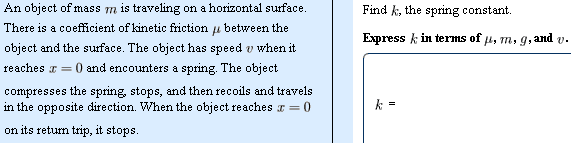# I am having some trouble with conservation of energy

## Homework Statement## Homework Equations

$$K_{1}$$+$$U_{1}$$+$$W_{nc}$$=$$K_{2}$$+$$U_{2}$$

## The Attempt at a Solution

Using the above equation

1/2m$$v^{2}$$ + 0 + xmg$$\mu$$ = 0 + 1/2k$$x^{2}$$

On the left side
When it hits the spring there is 0 potential because it has not yet compress the spring and it still remains on a horizontal surface. It has a kinetic energy and kinetic friction work
On the right side
I have 0 kinetic because it just finished compressing the spring and about to decompress so instead I get maximum potential energy.

So solving for K i get

$$\frac{mv^{2}+xmg\mu}{x^{2}}$$

But according to the answer it does not depend on the variable X (distance compressed by spring)
So I'm not entirely sure I know where I made my mistake

Last edited: